# Monotone 3-Satisfiability

I told Daniel when he gave me his Monotone Satisfiability reduction that the actual problem mentioned in G&J was Monotone 3-Satisfiability.  So he went off and did that reduction too.
The Problem:
Monotone 3 SAT. This is a more restrictive case of Monotone SAT

The Description:
Given an formula of clauses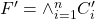where each clause in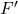contains all negated or non-negated variables, and each clause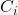contains at most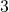variables. Does there exist an assignment of the variables so thatis satisfied?

Example: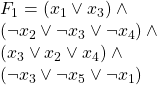the following assignment satisfies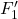: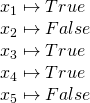However: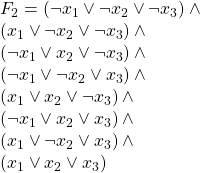And the following is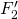in Monotone  3SAT form: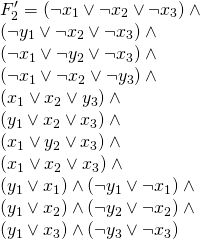are both unsatisfiable.

The reduction:
In the following reduction we are given an instance of 3SAT,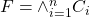. Here each clause is of the form: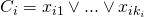where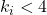and each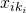is a literal of the form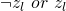.
We use the following construction to build an instance of Monotone  3 SAT out of the above instance of 3SAT :
In each clausewe have at most one literal,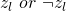that is not of the same parity as the rest of the literals in the clause. For every such literal, we may preform the following substitution: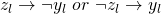this yields a modified clause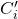.
Now we must be able to guarantee that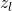and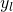are mapped to opposite truth values, so we introduce the new clause: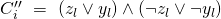and conjunct it onto our old formula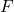producing a new formula.

For example: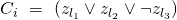so we preform the substitution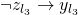so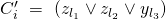and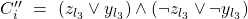Now repeating this procedure will result in a new formula: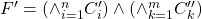.
We claim logical equivalence between the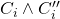and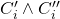This is semantically intuitive as the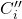clause requires all substituted literalinto take the value opposite ofthis was the stipulation for the substitution initially. It is also verifiable by truth table construction for: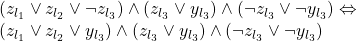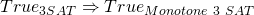:
If there exists a truth assignment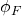that satisfies, then we may extent this truth assignment to produce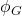which will satisfy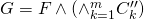by letting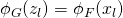for alland letting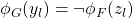for all.
Obviously ifis satisfiable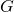must be by the above construction of. So by the above claim we have thatwill satisfy.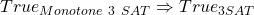:
Continuing from the above, if we have a truth assignment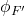that satisfies, then by the claim above it also must satisfy. Andis a sub-formula ofso any truth assignment that satisfiesmust also satisfy.

(Back to me)

Difficulty: 4, since it’s a little harder than the regular Monotone Sat one.

### 2 responses to “Monotone 3-Satisfiability”

1.Guest

Is this even more restrictive version of Monotone 3-SAT (Monotone 2-3 SAT) too NP-Complete:
1. All Clauses are monotone.
2. All positive monotone clauses are of length 3.
3. All negative monotone clauses are of length 2.

•Sean T. McCulloch

I don’t know, I haven’t heard of that variant. Let’s think about it.

The reduction above gets you most of the way to where you want to go, with 2 exceptions:
1) The transformation can create a positive monotone clause of length 2
2) The original F may have a negative monotone clause of length 3.

Of these, the second one worries me the most. Since 2-SAT is polynomial, we know that there isn’t a way to (in polynomial time) convert a set of clauses of length 3 into an equivalent set of clauses of length 2. (Because if we could, we’d have a reduction from 3SAT into 2SAT and P=NP). It seems like you’d have to do something like that to make this case work.

So my gut feeling is that it’s not NP-Complete. but it’s possible that there is a different clever reduction out there that would do it.